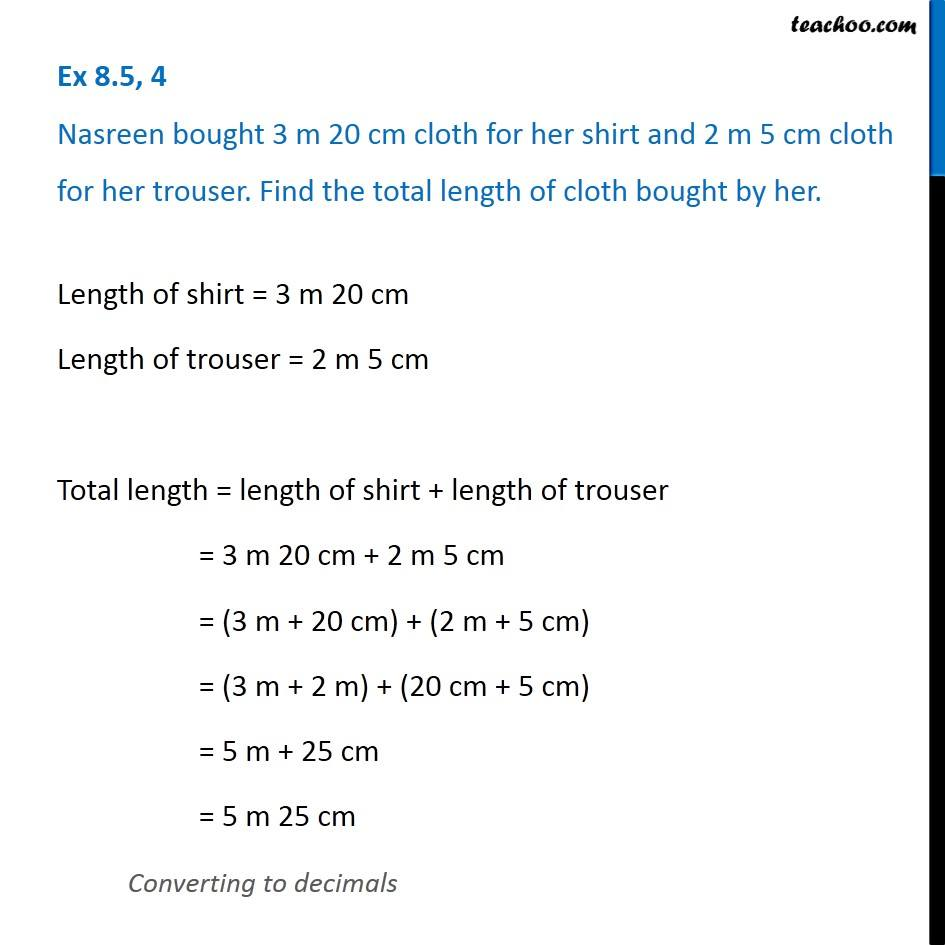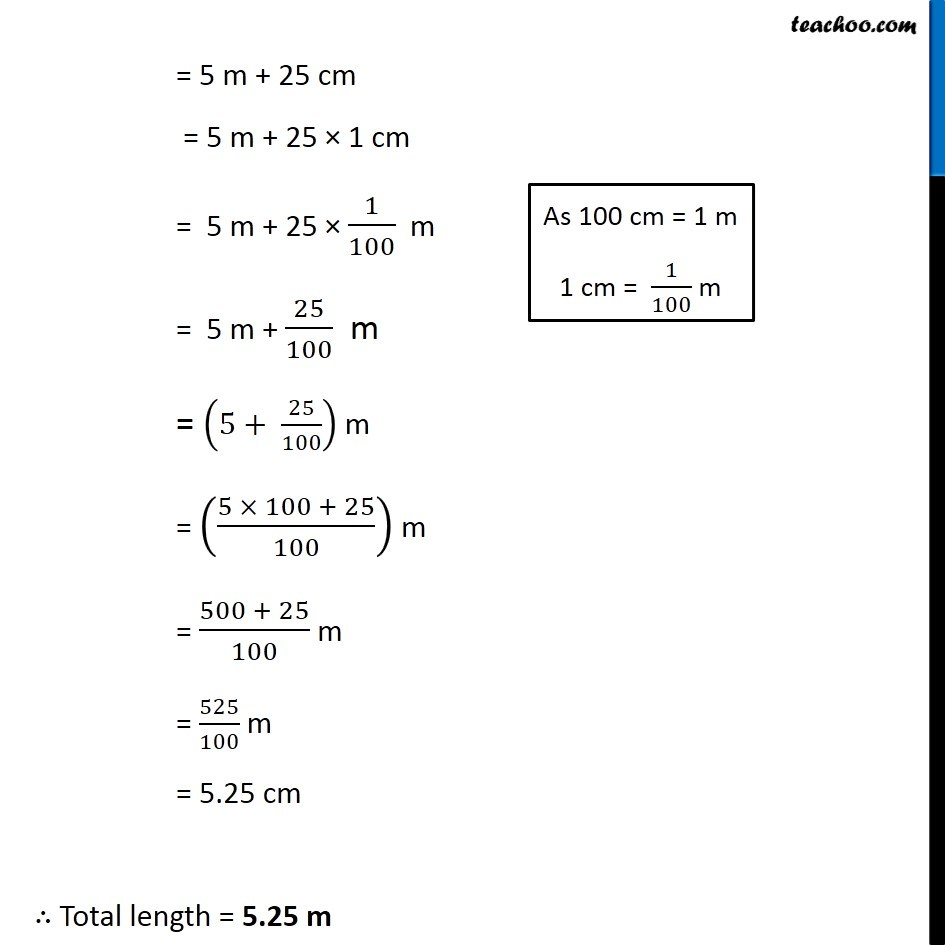Ex 8.5

Chapter 8 Class 6 Decimals
Serial order wiseGet live Maths 1-on-1 Classs - Class 6 to 12

### Transcript

Ex 8.5, 4 Nasreen bought 3 m 20 cm cloth for her shirt and 2 m 5 cm cloth for her trouser. Find the total length of cloth bought by her. Length of shirt = 3 m 20 cm Length of trouser = 2 m 5 cm Total length = length of shirt + length of trouser = 3 m 20 cm + 2 m 5 cm = (3 m + 20 cm) + (2 m + 5 cm) = (3 m + 2 m) + (20 cm + 5 cm) = 5 m + 25 cm = 5 m 25 cm Converting to decimals = 5 m + 25 cm = 5 m + 25 × 1 cm = 5 m + 25 × 1/100 m = 5 m + 25/100 m = (5+ 25/100) m = ((5 × 100 + 25)/100) m = (500 + 25)/100 m = 525/100 m = 5.25 cm ∴ Total length = 5.25 m As 100 cm = 1 m 1 cm = 1/100 m